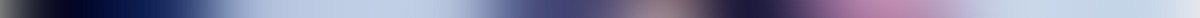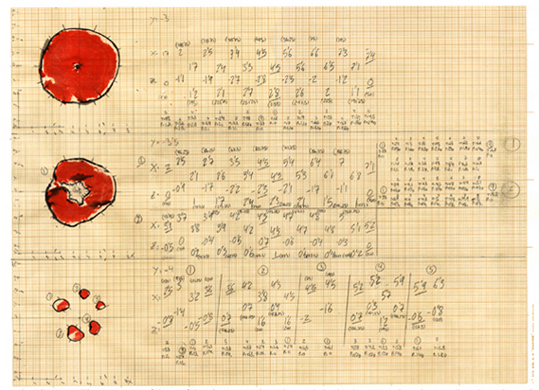# INFO

Each one of the 365 vertexes that define the volume of the apple has three values (X, Y and Z) depending on its relative position to the center of the apple. Depending on the direction in which the array of those dots is being performed, X, Y and Z values would correspond to Pitch, Panoramic, Volume or time values respectively. Once a unique sound is assigned to each one of the vertexes, they are “sound scanned” with a beam of light.

This project started long before the 3D scanners were invented so in order to create the virtual shape of the apple in the computer, the apple had to be sliced in sixteen sections, each section containing sixteen points. To get the X and Y values of those points each section was imprinted on grid millimeter paper using an special ink. The Z value was defined by the slice number.The Apple is defined by 365 vertexes, each vertex has three values (X, Y and Z)Three of the sixteen imprints on millimeter paper used to make the 3D model